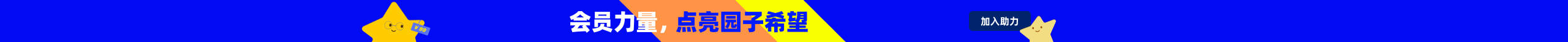# 每个人都应该懂点函数式编程

//绘制直线函数图像
public void DrawLine(double a, double b)
{
List<PointF> points = new List<PointF>();
for(double x=-10;x<=10;x=x+0.1)
{
PointF p =new PointF(x,a*x+b);
}
//将points点连接起来
}
//绘制抛物线图像
public void DrawParabola(double a, double b, double c)
{
List<PointF> points = new List<PointF>();
for(double x=-10;x<=10;x=x+0.1)
{
PointF p =new PointF(x,a*Math.Pow(x,2) + b*x + c);
}
//将points点连接起来
}
...
DrawLine(3, 4);   //绘制直线
DrawParabola(1, 2, 3);    //绘制抛物线

（注，如果采用虚方法的方式，要绘制N种不同函数图像就需要定义N个类，每个类中都需要重写生成points的算法）

public delegate double Function2BeDrawed(double x);
//绘制函数图像
public void DrawFunction(Function2BeDrawed func)
{
List<PointF> points = new List<PointF>();
for(double x=-10;x<=10;x=x+0.1)
{
PointF p =new PointF(x,func(x));
}
//将points点连接起来
}
...
Function2BeDrawed func =
(Function2BeDrawed)((x) => { return 3*x + 4;}); //创建直线函数
DrawFunction(func);  //绘制系数为3、4的直线
Function2BeDrawed func2 =
(Function2BeDrawed)((x) => {return 1*Math.Pow(x,2) + 2*x + 3;}); //创建抛物线函数
DrawFunction(func2);  //绘制系数为1、2、3的抛物线
Function2BeDrawed func3 =
(Function2BeDrawed)((x) => {return 3*Math.Sin(x) + 4;}); //创建正弦函数
DrawFunction(func3);  //绘制系数为3、4的正弦函数图像

int x = 1;

let func x y = x + y

int x = 1;
int y = x;

let func = fun x y -> x + y  //lambda表达式
let func2 = func

int[] ints = new int[]{1, 2, 3, 4, 5};

let func x = x + 1
let func2 x = x * x
let func3 = fun x -> x - 1    //lambda表达式
let funcs = [func; func2; func3]  //存入列表，注意存入列表的函数签名要一致

void func(int a, int b)
{
    //
}
func(1, 2);

let func x = x * x  //定义函数func
let func2 f x =   //定义函数func2 第一个参数是一个函数
   f x
func2 func 100   //将func和100作为参数 调用func2

int func(int x)
{
    return x + 100;
}
int result = func(1);  //result为101

let func x =
   let func2 = fun y -> x + y
   func2             //将函数func2作为返回值
let result = (func 100) 1  //result为101，括号可以去掉

1.函数定义

2.无副作用

3.柯里化

let func x y = x + y
let result = func 1 2  //result为3

let func x =
   let func2 = fun y -> x + y
   func2
let result = (func 1) 2   //result结果也为3，可以去掉括号

4.不可变性

5.高阶函数

foreach(Person p in list)
{
    if(p.Age > 25)
    {
        //...
    }
}

list.Where(p => p.Age>25).Select(p => p.Name).toArray();

posted @ 2015-08-27 11:47  周见智  阅读(16595)  评论(19编辑  收藏  举报